Get instant live expert help with Excel or Google Sheets“My Excelchat expert helped me in less than 20 minutes, saving me what would have been 5 hours of work!”

#### Post your problem and you’ll get Expert help in seconds.

Your message must be at least 40 characters
Our professional Expert are available now. Your privacy is guaranteed.

# How to Get the Maximum in Excel IF There Are Multiple Criteria

We can get the maximum value of a dataset without considering any criteria by simply using the MAX or LARGE function in Excel. But what if we need to consider one or multiple criteria to get the maximum value? In this article, we will learn how to get the maximum if multiple criteria by using different methods.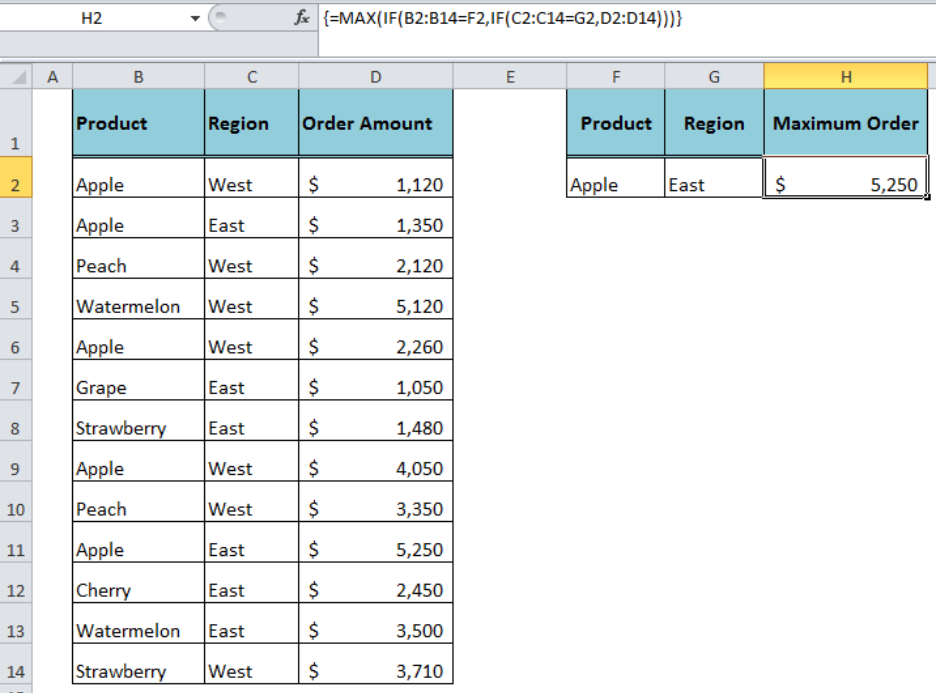Figure 1. How to Get Maximum If Multiple Criteria

## Array Formula Approach

We can get the maximum if multiple criteria value of a dataset with the help of array formula approach based on MAX or LARGE function along with IF function in Excel. The array formula must be entered using Ctrl+Shift+Enter keyboard keys, and as a result of this operation, the formula is automatically surrounded by curly brackets ({ }).

### Using MAX and IF Functions

We have a dataset of orders for multiple products in different regions and we need to get the maximum order amount of a given product in a given region. Using an array formula based on MAX and IF functions we can get the maximum if multiple criteria in the following formula:

`=MAX(IF(B2:B14=F2,IF(C2:C14=G2,D2:D14)))`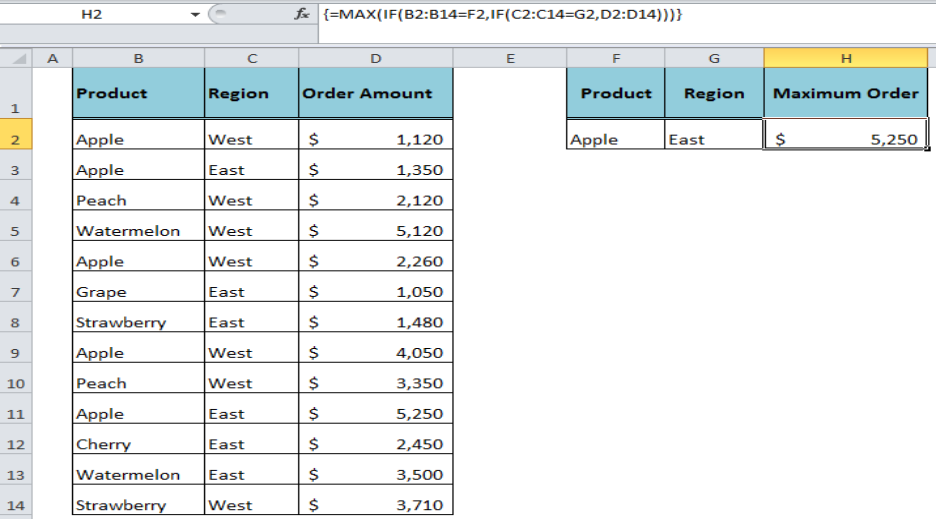Figure 2. Using MAX and IF Functions

### Using LARGE and IF Functions

Using an array formula based on LARGE and IF functions we can get the maximum order amount if there are multiple criteria in the following formula:

`=LARGE(IF(B2:B14=F2,IF(C2:C14=G2,D2:D14)),1)`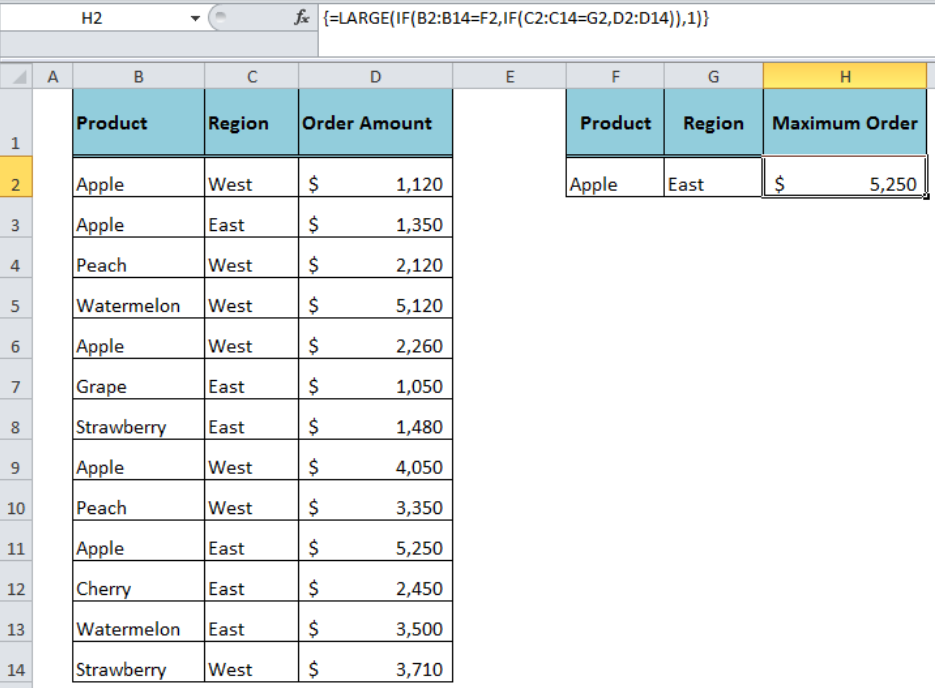Figure 3. Using LARGE and IF Functions

## Non-Array Formula Approach

Using special functions that natively handle the arrays we can get the maximum if multiple criteria in Excel, such as MAXIFS and SUMPRODUCT functions.

### Using the MAXIFS Function

The MAXIFS function has been introduced in Excel 2016 to return the maximum value based on one or multiple criteria without using an array formula. Using the following MAXIFS formula we can get the maximum order amount for “Apple” in “East” region:

`=MAXIFS(D2:D14,B2:B14,F2,C2:C14,G2)`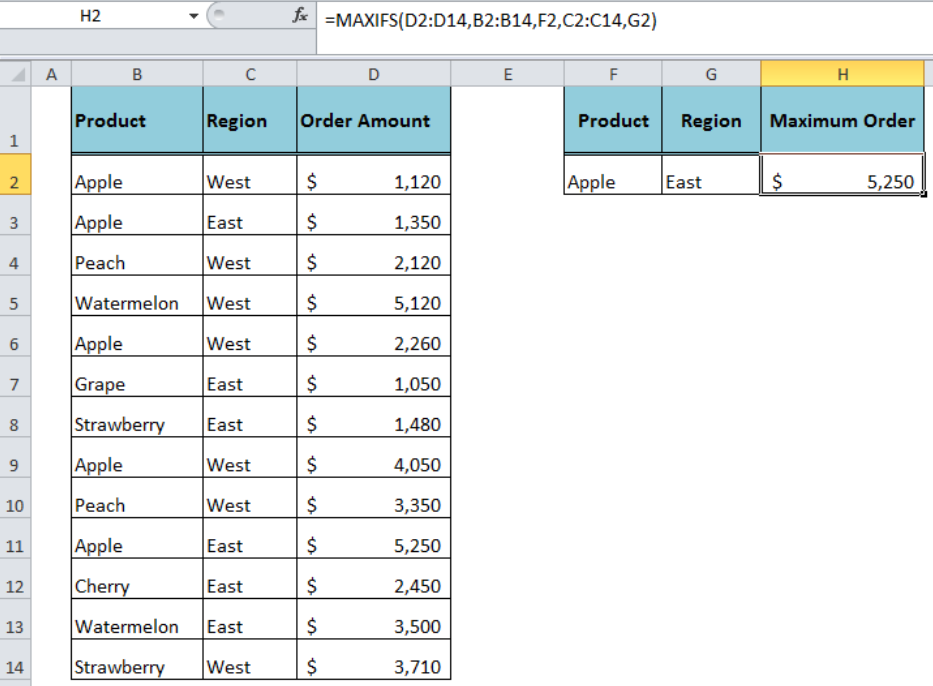Figure 4. Using the MAXIFS Function

### Using SUMPRODUCT and MAX Functions

As the SUMPRODUCT function handles the arrays natively, so we can use this function along with MAX function to get the maximum if multiple criteria in Excel 2013 and earlier versions in the following formula:

`=SUMPRODUCT(MAX((B2:B14=F2)*(C2:C14=G2)*(D2:D14)))`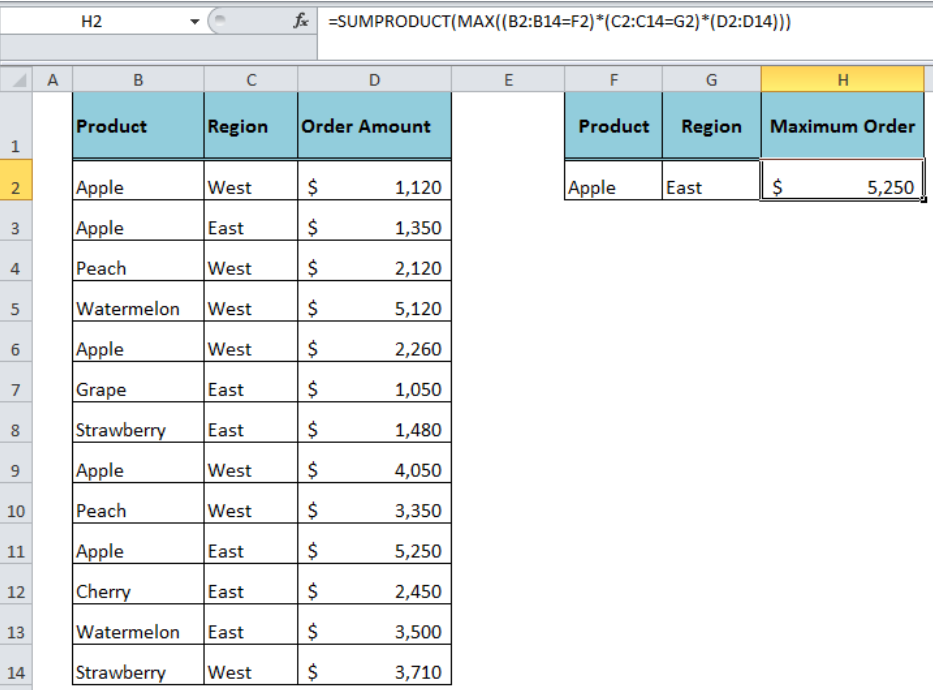Figure 5. Using SUMPRODUCT and MAX Functions

### Using SUMPRODUCT and LARGE Functions

Using SUMPRODUCT and LARGE functions we can get the maximum value of a dataset based on multiple criteria in the following non-array formula:

`=SUMPRODUCT(LARGE((B2:B14=F2)*(C2:C14=G2)*(D2:D14),1))`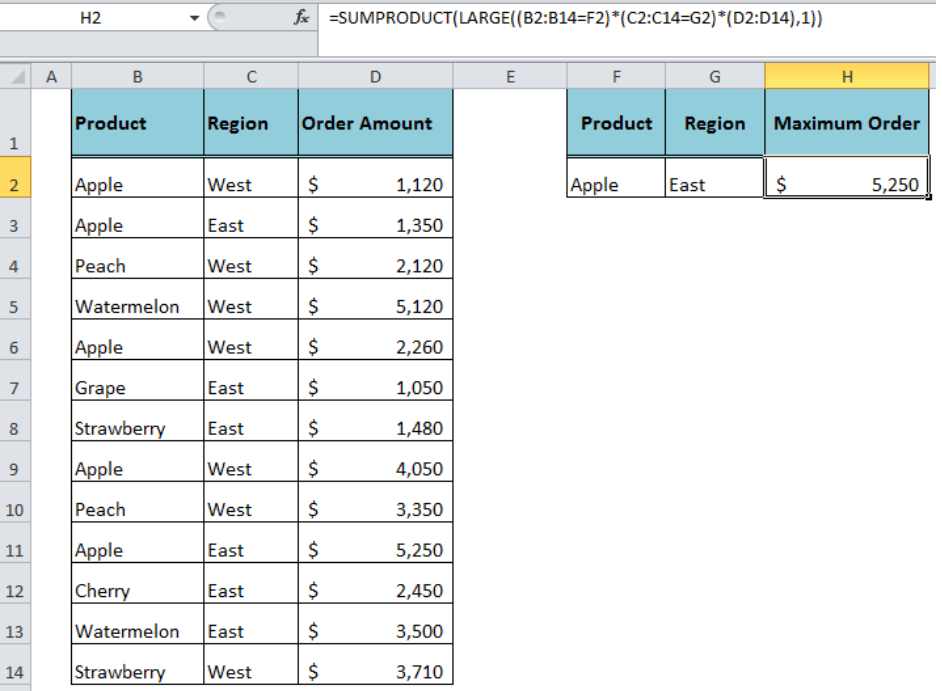Figure 6. Using SUMPRODUCT and LARGE Functions

## Instant Connection to an Expert through our Excelchat Service

Most of the time, the problem you will need to solve will be more complex than a simple application of a formula or function. If you want to save hours of research and frustration, try our live Excelchat service! Our Excel Experts are available 24/7 to answer any Excel question you may have. We guarantee a connection within 30 seconds and a customized solution within 20 minutes.

### Did this post not answer your question? Get a solution from connecting with the expert.Another blog reader asked this question today on Excelchat:
Solution examplesI need cell B2 Green if cells G2:Q2 are empty. If cells G2:Q2 have any text in any cell then i need B2 to be red.
Solved by C. W. in 20 minsI have a list of cost prices in one column and i would like to know what formula to use to add a percentage value. E.g if my cost price is just Â£1 i would like to create the sell price to be perhaps Â£3, but if my cost price is Â£1000, i would like the sell price to perhaps be Â£1,100 (not Â£3,000).
Solved by M. Y. in 60 minshello i need help creating a formula, if a value does not exsits put 0, if a value exist do nothing
Solved by M. F. in 9 minsCompute the weighted average of students score from a spreadsheet using different weight for each category of marks
Solved by G. E. in 20 minshi there, im making a spread sheet to track progress in a game and would like to create a drop log sheet, i was hoping to use the if function to detect weather a item is logged on a sheet then send the sheet number to a list if so
Solved by K. F. in 60 mins## Subscribe to Excelchat.coAnother blog reader asked this question today on Excelchat: